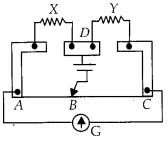Enlightened

# Question 10: NCERT Solutions for 12th Class Physics: Chapter 3-Current Electricity

• 0

Question 10: NCERT Solutions for 12th Class Physics: Chapter 3-Current Electricity

(a) In a meter bridge as shown in figure, the balance point is found to be at 39.5 cm from the end A, when the resistor is of 12.5 Ω. Determine the resistance of x. Why are the connection between resistors in a Wheatstone or meter bridge made of thick copper strips?(b) Determine the balance point of the bridge above if x and are interchanged.
(c) What happens if the galvanometer and cell are interchanged at the balance point of the bridge? Would the galvanometer show any current?

Share

1. Solution:
(a)The connections are made of thick copper strips so as to provide negligible resistance by connecting wires.
(b) If x and Y are interchanged the balance point will be at 60.5 cm from A.
(c) In balanced condition of the bridge, the cell and the galvanometer can be exchanged, the galvanometer will still show zero deflection.Check the complete chapter with solutions.

NCERT Solutions for 12th Class Physics: Chapter 3-Current Electricity

• 0